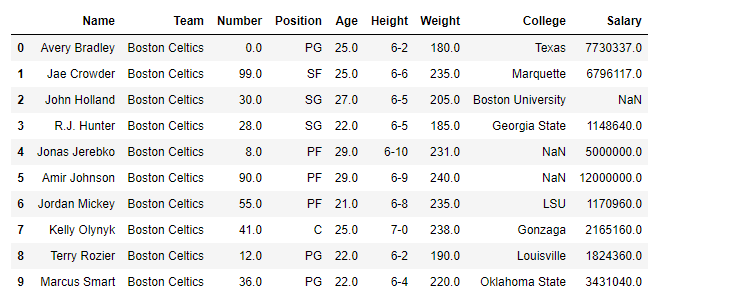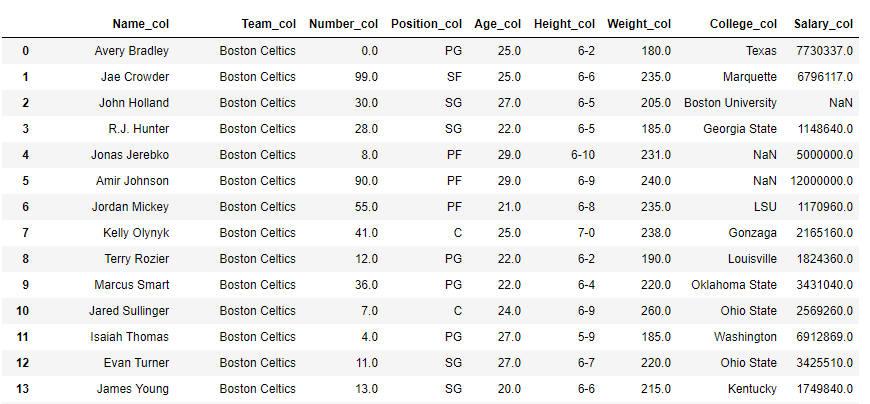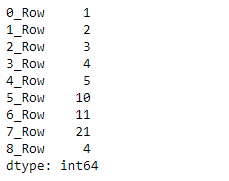Python is a great language for doing data analysis, primarily because of the fantastic ecosystem of data-centric python packages. Pandas is one of those packages and makes importing and analyzing data much easier.
`Dataframe.add_suffix()` function can be used with both series as well as dataframes. `add_suffix()` function Concatenate suffix string with panel items names.

• For Series, the row labels are suffixed.
• For DataFrame, the column labels are suffixed.
```Syntax: DataFrame.add_suffix(suffix)

Parameters:
suffix : string

Returns:  with_suffix: type of caller
```

Example #1: Suffix `_col `in each columns in the dataframe.

 `# importing pandas as pd ` `import` `pandas as pd ` ` `  `# Making data frame from the csv file ` `df ``=` `pd.read_csv(``"nba.csv"``) ` ` `  `# Printing the first 10 rows of ` `# the data frame for visualization ` `df[:``10``] ``# Using add_suffix() function to  ` `# add '_col' in each column label ` `df ``=` `df.add_suffix(``'_col'``) ` ` `  `# Print the dataframe ` `df  `

Output:Example #2: Using `add_suffix()` with Series in pandas

`add_suffix()` alters the row index labels in the case of series.

 `# importing pandas as pd ` `import` `pandas as pd ` ` `  `# Creating a Series  ` `df ``=` `pd.Series([``1``, ``2``, ``3``, ``4``, ``5``, ``10``, ``11``, ``21``, ``4``]) ` ` `  `# This will suffix '_Row' in ` `# each row of the series ` `df ``=` `df.add_suffix(``'_Row'``) ` ` `  `# Print the Series ` `df `

Output:My Personal Notes arrow_drop_upCheck out this Author's contributed articles.

If you like GeeksforGeeks and would like to contribute, you can also write an article using contribute.geeksforgeeks.org or mail your article to contribute@geeksforgeeks.org. See your article appearing on the GeeksforGeeks main page and help other Geeks.

Please Improve this article if you find anything incorrect by clicking on the "Improve Article" button below.

Article Tags :

Be the First to upvote.

Please write to us at contribute@geeksforgeeks.org to report any issue with the above content.